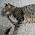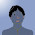## 2018年11月17日 星期六

### 因為無知，所以出糗--淺談彩虹表原理

(摘自TWWORD的「彩虹表」詞條）

• 雜湊函式將明文密碼轉換成雜湊值，用 H( ) 代替。
• 歸約函式將雜湊值對應到某個明文密碼，用 R( ) 代替。

Ha = H(A) , Hb = H(B), ... ..., Hz = H(Z)   # Ha 即明文密碼A經過雜湊後的結果。

 Rm = R(Ha)=M Rl = R(Hb)=L Rg = R(Hc)=G Ro = R(Hd)=O Rp = R(He)=Q Rc = R(Hf)=C Rr = R(Hg)=R Rd = R(Hh)=D Rn = R(Hi)=N Rx = R(Hj)=X Re = R(Hk)=E Rs = R(Hl)=S Rf = R(Hm)=F Rj = R(Hn)=J Rk = R(Ho)=K Ru = R(Hp)=U Rv = R(Hq)=V Ri = R(Hr)=I Rq = R(Hs)=Q Rb = R(Ht)=B Rh = R(Hu)=H Rw = R(Hv)=W Rt = R(Hw)=T Ra = R(Hx)=A Rp = R(Hy)=P Rd = R(Hz)=D

1. 從明文密碼中隨機選擇一項(假設為 M），由雜湊函式計算其雜湊值得到Hm
2. Hm送給歸約函式計算，反轉得到 F
3. 再將F交給雜湊函式，又得一組雜湊值Hf
4. 再將Hf送給歸約函式計算，反轉得到 C

 M H() → Hm R() → F H() → Hf R() → C H() → Hc R() → G I H() → Hi R() → N H() → Hn R() → J H() → Hj R() → X Q H() → Hq R() → V H() → Hv R() → W H() → Hw R() → T D H() → Hd R() → O H() → Ho R() → K H() → Hk R() → E
~~底下還有，省略~~

 M H() → Hm R() → F H() → Hf R() → C H() → Hc R() → G H() → Hg R() → R H() → Hr R() → I H() → Hi R() → N H() → Hn R() → J K H() → Hk R() → E H() → He R() → Q H() → Hq R() → V H() → Hv R() → W H() → Hw R() → T H() → Ht R() → B H() → Hb R() → L P H() → Hp R() → U H() → Hu R() → H H() → Hh R() → D H() → Hd R() → O H() → Ho R() → K H() → Hk R() → E H() → He R() → Q
~~底下還有，省略~~

1. Hw歸約函式得到T
2. 比對每條彩虹鍵的尾端，在第三條鏈找到T，因而得知該鏈的頭是Q
3. Q計算得雜湊HqHq≠Hw
4. Hq歸約函式計算得V，用V計算得雜湊HvHv≠Hw
5. Hv歸約函式計算得W，用W計算得雜湊HwHw=Hw，所以求得答案為W

1. Hw歸約函式得到T
2. T比對每條彩虹鍵的尾端，都沒有找到T
3. T雜湊Ht，再將Ht歸約B
4. B 比對每條彩虹鍵的尾端，都沒有找到B
5. B雜湊Hb，再將Hb歸約L
6. L比對每條彩虹鍵的尾端，在第二條鏈找到L，因而得知該鏈的頭是K
7. K計算得雜湊HkHk≠Hw
8. Hk歸約函式計算得E，用E計算得雜湊HeHe≠Hw
9. He歸約函式計算得Q，用Q計算得雜湊HqHq≠Hw
10. Hq歸約函式計算得V，用V計算得雜湊HvHv≠Hw
11. Hv歸約函式計算得W，用W計算得雜湊HwHw=Hw ，所以求得答案為W

#### 2 則留言:

1.實作可參考python開源https://github.com/jfmengels/rainbowtable-python

1.感謝您提供的資訊，謝謝!